GRAPHS OF SOME INTERESTING POLAR EQUATIONS

Example 1:

Go to desmos.com and in settings change from the rectangular to the polar grid. You must useon the desmos keyboard! Type r = 2 cos 3and observe the graph.

The graph is a Rose Curve with 3 petals since 3 is odd.Example 2:

Go to desmos.com and in settings change from the rectangular to the polar grid. You must useon the desmos keyboard! Type r = 2 cos 2and observe the graph.

The graph is a Rose Curve with 4 petals since 2 is even.Example 3:

Go to desmos.com and in settings change from the rectangular to the polar grid. You must useon the desmos keyboard! Type r = 1 + cosand observe the graph.

The graph is a heart-shaped Limacon also know as a Cardiod.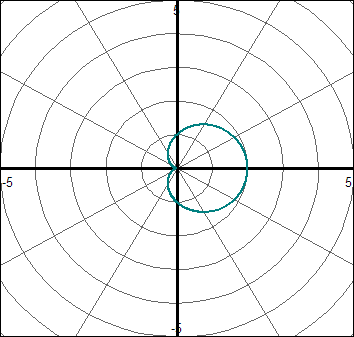Example 4:

Go to desmos.com and in settings change from the rectangular to the polar grid. You must useon the desmos keyboard! Type r = 1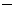2sinand observe the graph.

The graph is a Limaçon with inner loop. Please note that.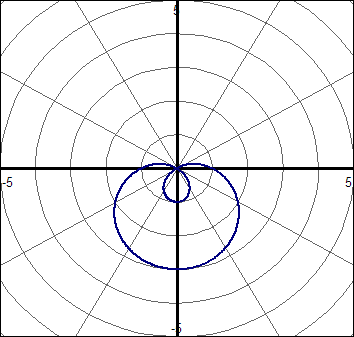Example 5:

Name the following limaçons:

a.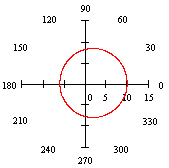r = 8 + 2 cosConvex Limaçon because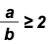.

b.r = 3 + 2 sinDimpled Limaçon because.

c.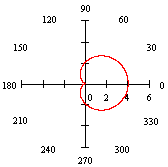r = 2 + 2 cosHeart-Shaped Limaçon (Cardiod) because.

d.r = 2 + 3cosLimaçon with Inner Loop because.

6. Find the number of petals of the Rose Curve with equation r = 2 sin (3).

The angle multiplier n = 4 is odd, therefore, this rose curve has 3 petals.

7. Find the number of petals of the Rose Curve with equation r = 2 cos (4) .

The angle multiplier n = 4 is even, therefore, this rose curve has 2(4) = 8 petals.

8. Give the equation of each circle graph with its equation.

a.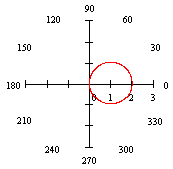This is a circle with center along the polar axis. It has a radius of length 1. Therefore, its equation must be r = 2 cos.

b.This is a circle with center at the pole. It has a radius of length 2. Therefore, its equation must be.

c.This is a circle with center along the r-axis. It has radius of length 1.5. Therefore, its equation must be r = 3 sin.

9. Give the radius of each circle.

a. r = cosThis is a circle with center along the polar axis. Here, a = 1. It has a radius of length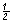.

b. r = 10 cosThis is a circle with center along the r-axis. Here, a = 10. It has a radius of lengthwhich is 5.

c. r = 12

This is a circle with center at the pole. Here, a = 12. It has a radius of length 12.